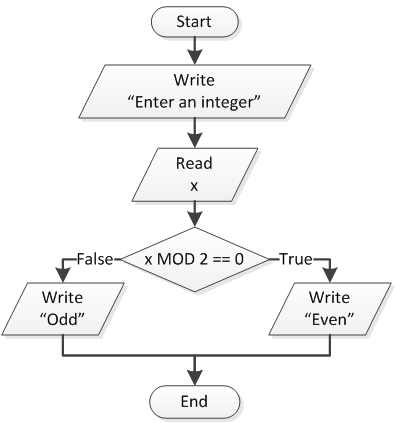Design a flowchart and write the corresponding program that prompts the user to enter an integer, and then displays a message indicating whether this number is odd or even.

###### Solution

In this exercise, you need to find a way to determine whether a number is odd or even. You need to find a common attribute between all even numbers, or between all odd numbers. And actually there is one! All even numbers are exactly divisible by 2. So, when the result of the operation `X MOD 2` equals 0 (zero), `X` is even; otherwise, `X` is odd.

Next you can find various odd and even numbers:

• Odd numbers: 1, 3, 5, 7, 9, 11, …
• Even numbers: 0, 2, 4, 6, 8, 10, 12, ….

Notice: Please note that zero is considered an even number.

The flowchart is shown here.and the program is shown here.

## PHP

```<?php
echo "Enter an integer: ";
\$x = trim(fgets(STDIN));

if (\$x % 2 == 0) {
echo "Even";
}
else {
echo "Odd";
}
?>
```

## Java

```public static void main(String[] args) throws java.io.IOException {
int x;

System.out.print("Enter an integer: ");

if (x % 2 == 0) {
System.out.println("Even");
}
else {
System.out.println("Odd");
}
}
```

## C++

```#include <iostream>
using namespace std;
int main() {
int x;

cout << "Enter an integer: ";
cin >> x;

if (x % 2 == 0) {
cout << "Even" << endl;
}
else {
cout << "Odd" << endl;
}
return 0;
}
```

## C#

```static void Main() {
int x;

Console.Write("Enter an integer: ");

if (x % 2 == 0) {
Console.WriteLine("Even");
}
else {
Console.WriteLine("Odd");
}

}
```

## Visual Basic

```Sub Main()
Dim x As Integer

Console.Write("Enter an integer: ")

If x Mod 2 = 0 Then
Console.WriteLine("Even")
Else
Console.WriteLine("Odd")
End If

```x = int(input("Enter an integer: "))